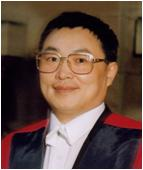﻿ 杨新社-西安工程大学理学院首 页> 人才培养> 研究生教育> 导师介绍

## 杨新社1. Yang X.-S., Engineering Optimization: An Introduction with Metaheuristic Applications,John Wiley and Sons, (2010).

2. Yang X.-S. and Koziel S., Computational Optimization and Applications in Engineering and Industry, Springer, (2011).

3. Yang X. S., Nature-Inspired Metaheuristic Algorithms, Luniver Presss, 2nd Edition (2010),[First Edition, 2008].

4. Yang X.-S. Introduction to Computational Mathematics, World Scientific, (2008) [Best-seller].

5. Yang X.-S., Introduction to Mathematical Optimization: From Linear Programming to Metaheuristic, Cambridge Int. Science Publishing, 2008.

6. Yang X. S., Metaheuristic optimization: algorithm analysis and open problems,Experimental Algorithms, Lecture Notes in Computer Sciences, 6630, pp. 21-32 (2011).

7. Yang X. S., Firefly Algorithm, Stochastic Test Functions and Design Optimisation, Int. J.Bio-Inspired Computation, Vol. 2, No. 2, pp.78-84 (2010).

8. Yang X. S. and Koziel S., Computational optimization, modelling and simulation – a paradigm shift, Procedia in Computer Science, 1, 1291-1294 (2010).

9. Yang X. S. and Deb S., Engineering optimization by cuckoo search, Int. J.Math. Mod. Num. Opt., Vol. 1, 330-343(2010).

10. Yang X. S. Modelling heat transfer of carbon nanotubes, Modelling and Simulation in Materials Science and Engineering, Vol.13, 893-903 (2005).